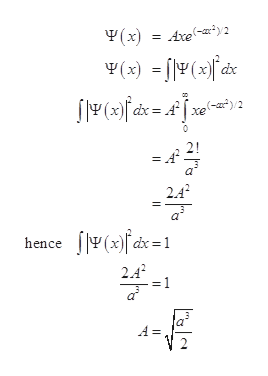# The wavefunction of is Ψ(x) = Axe(−ax^2)/2 for with energy E = 3aℏ2/2m. Find the bounding potential V(x). Looking at the potential’s form, can you write down the two energy levels that are immediately above E?

Question
212 views

The wavefunction of is Ψ(x) = Axe(−ax^2)/2
for with energy E = 3aℏ2/2m.
Find the bounding potential V(x). Looking at the potential’s form, can you write down the two energy levels that are immediately above E?

check_circle

Step 1

Given wave function of is Ψ(x) = Axe (−ax^2)/2

Step 2

To determine the normali...help_outlineImage TranscriptioncloseY (x) = Axe*y2 ¥(x) = [|Y(x)* äx dx = 4[xe-z)/2 2! 2.4 f|Y(x)/ dx = 1 hence 2.4 || fullscreen

### Want to see the full answer?

See Solution

#### Want to see this answer and more?

Solutions are written by subject experts who are available 24/7. Questions are typically answered within 1 hour.*

See Solution
*Response times may vary by subject and question.
Tagged in

### Other